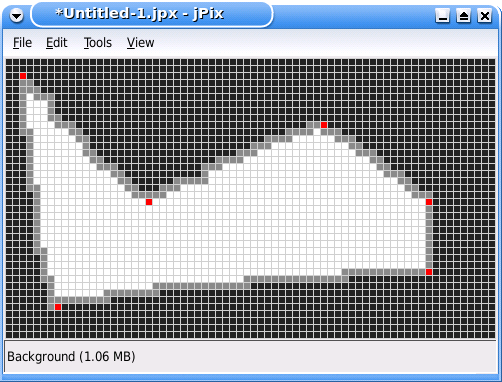# Internet Problem Solving Contest

## Problem J – jPix

jPix is a new, very promising, open source bitmap editor. As the project is in a very alpha stage of development, currently jPix supports only 3 colors: white, grey and black. Other features of jPix include drawing straight lines with grey color and floodfill with black color. However, the most promising and coolest feature of jPix is the ability to work with images of vast sizes.

To draw lines jPix uses the following advanced algorithm:

```void draw_line(int x1, int y1, int x2, int y2)
{
int x_middle = (x1+x2)/2;
int y_middle = (y1+y2)/2;

set_color(GREY);
put_pixel(x_middle, y_middle);

if((x1!=x2) || (y1!=y2))
{
if(abs(x1-x2) > abs(y1-y2))
{
if(x1<x2)
{
draw_line(x1, y1, x_middle, y_middle);
draw_line(x_middle+1, y_middle, x2, y2);
}
else // x1>x2
{
draw_line(x1, y1, x_middle+1, y_middle);
draw_line(x_middle, y_middle, x2, y2);
}
}
else
{
if(y1<y2)
{
draw_line(x1, y1, x_middle, y_middle);
draw_line(x_middle, y_middle+1, x2, y2);
}
else // y1>y2
{
draw_line(x1, y1, x_middle, y_middle+1);
draw_line(x_middle, y_middle, x2, y2);
}
}
}
}
```

One of the features that is urgently needed among its users is the ability to report the number of pixels of each color in the image. The developers of jPix, unable to come up with an efficient solution, asked us, the IPSC team, for help. Since we are lost too, we ask you to help us.

### Problem specification

A jPix image is X pixels wide and Y pixels high. The pixel (0,0) is the upper left corner, and the pixel (X-1,Y-1) is the lower right corner. When jPix starts, the image is completely white.

The user is allowed to draw several line segments using grey color. The segments, however, must form a simple polygon. (See the input specification for a more precise definition.) Then the user must issue the flood fill command, starting from pixel (0,0); the fill color is black. (Since jPix is quite buggy, any other sequence of commands crashes jPix.)

Given the list of the commands issued by the user, your task is to implement the requested feature which will count the number of pixels of each color.

### Input specification

The first line of the input file contains an integer T specifying the number of test cases. Each test case is preceded by a blank line.

Each test case looks as follows: The first line contains two positive integers X and Y, the width and height of the image. The following line contains N – the number of line segments drawn by the user. Each of the following N lines contains four positive integers x1, y1, x2, y2 describing the two endpoints (x1,y1) and (x2,y2) of a line segment. You may assume that the line segments are given in order and correctly describe a simple polygon which is not touching the border of the image.

More precisely, you may assume the following things:

• The exact mathematical object defined by the N line segments is a simple polygon, that is, any pair of consecutive edges has one point in common (the common vertex) and no pair of edges that are not consecutive has a point in common.
• The lines are given in the order in which they occur on the polygon's boundary. That is, the second line must start where the first ended, third must start where the second ended etc., and the last line must end where the first one started.
• After the lines are painted in jPix, no grey pixel will lie on the border of the image.

### Output specification

For each test case output a line with three positive integers – the number of pixels of white, grey, and black color, respectively.

### Example

Input:
```3

10 10
3
3 3 8 8
8 8 8 3
8 3 3 3

10 10
4
2 2 6 2
6 2 6 6
6 6 2 6
2 6 2 2

70 40
6
2  2  20 20
20 20 45 9
45 9  60 20
60 20 60 30
60 30 7  35
7  35 2  2
```
Output:
```7 20 73
9 16 75
931 202 1667
```The image shows a typical jPix session corresponding to the third example input. For your convenience the line endpoints are shown in red. In the actual image produced by jPix these points are grey.

Credits:
Problemsetter(s): misof